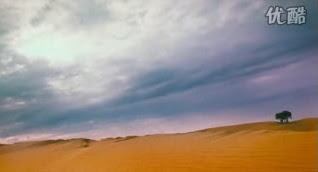Mar 14

## 黄色沙漠[simage=163,320,y,none,both]

[simage=164,320,y,none,both]

[simage=165,320,y,none,both]

[simage=166,320,y,none,both]

[simage=167,320,y,none,both]

[simage=168,320,y,none,both]

[simage=169,320,y,none,both]

[simage=170,320,y,none,both]

[simage=171,320,y,none,both]

[simage=172,320,y,none,both]

[simage=173,320,y,none,both]

[simage=174,320,y,none,both]

[simage=175,320,y,none,both]

[simage=176,320,y,none,both]

[simage=177,320,y,none,both]

[simage=178,320,y,none,both]

written by panhezeng \\ tags: